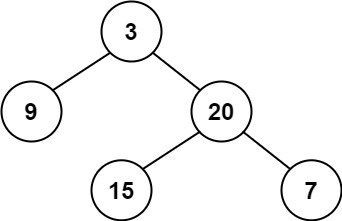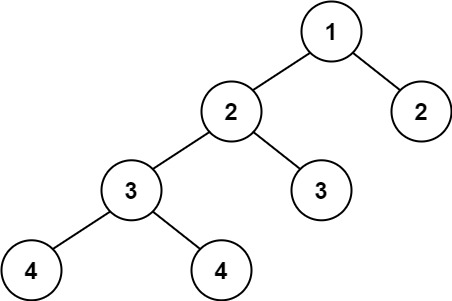# LeetCode: 110. Balanced Binary Tree

Go to the source link to view the article. You can view the picture content, updated content and better typesetting reading experience. If the link is broken, please click the button below to view the snapshot at that time.

## 题目¶

Given a binary tree, determine if it is height-balanced.

For this problem, a height-balanced binary tree is defined as:

a binary tree in which the left and right subtrees of every node differ in height by no more than 1.

Example 1:```Input: root = [3,9,20,null,null,15,7]
Output: true
```

Example 2:```Input: root = [1,2,2,3,3,null,null,4,4]
Output: false
```

Example 3:

```Input: root = []
Output: true
```

Constraints:

• The number of nodes in the tree is in the range [0, 5000].
• -104 <= Node.val <= 104

## 解法¶

```# Definition for a binary tree node.
# class TreeNode:
#     def __init__(self, val=0, left=None, right=None):
#         self.val = val
#         self.left = left
#         self.right = right
class Solution:
def isBalanced(self, root):
if root is None:
return True
left_height = self.max_height(root.left)
right_height = self.max_height(root.right)

return abs(left_height - right_height) <= 1 and self.isBalanced(root.left) and self.isBalanced(root.right)

def max_height(self, root):
if root is None:
return 0
return 1 + max(self.max_height(root.left), self.max_height(root.right))
```